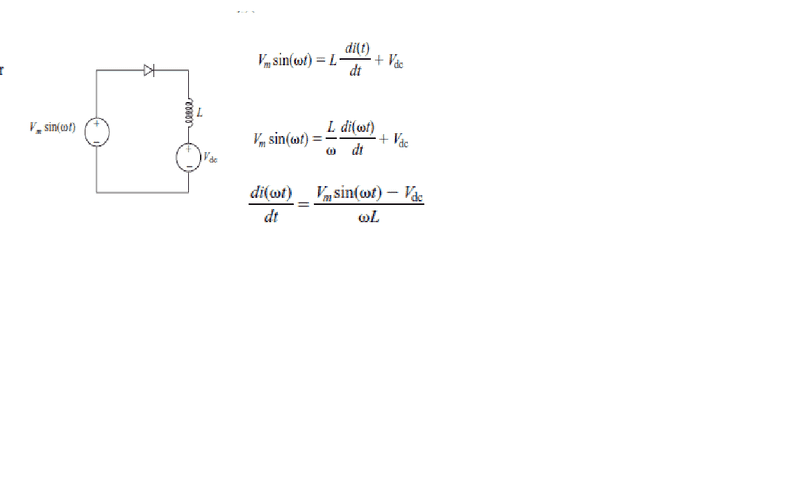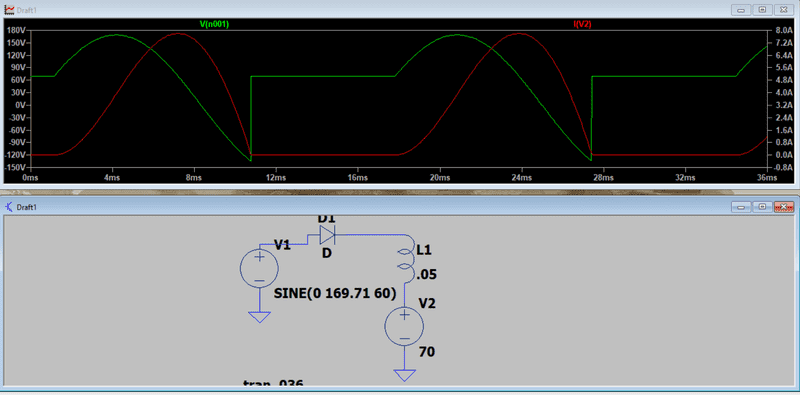# Trying to analyze a half wave rectifier with inductor and DC source

Jason06841
TL;DR Summary
I am trying to analyze a half wave rectifier with an inductor and DC source load.
I am trying to analyze a half wave rectifier with an inductor and DC source load. I understand the circuit but I guess I do not get the math. I am reading a book and this is the circuit and equations they came up with. I understand how they got from the first equation to the second equation but I do not understand their algebra to get from the second equation to the third. Shouldn't it be w/L instead of 1/wL? How do they get to the third equation?Gold Member
Looks as if the final equation is correct but I do not understand Eq 2.
It also looks to me that the current will not be sinusoidal, as the DC voltage biases the diode beyond cut off for part of the cycle.

•Jason06841 and DaveE
Staff Emeritus
TL;DR Summary: I am trying to analyze a half wave rectifier with an inductor and DC source load.

I am reading a book and this is the circuit and equations they came up with.

Jason06841
Looks as if the final equation is correct but I do not understand Eq 2.
It also looks to me that the current will not be sinusoidal, as the DC voltage biases the diode beyond cut off for part of the cycle.
These are just the equations for when the diode is forward biased. Starting from Vdc+ to partly into the negative half cycle due to the energy stored in L.

He does a few things there from equation 1 to equation 2. First he redefines the domain from t to wt. Because that would make the lower differential d(wt). But w is constant (in this case) so he is able to pull it out and multiply it by dt and continue to solve the equation in terms of t. However, that puts the w in the denominator so when you rearrange you get w/L instead of what he got which was 1/wL.

•DaveE
I am reading a book and this is the circuit and equations they came up with.
Some of us want to know the title, author and ISBN, so we can peruse that chapter.

It seems;
To get from eqn(1) to eqn(2), multiply the rhs by w/w = 1;
Then subtract Vdc from both sides of eqn(2).
Knowing; ZL = w⋅L ;
Eqn(3) is Ohms law, with i = v / ZL.

•Jason06841 and scottdave
Gold Member
Some of us want to know the title, author and ISBN, so we can peruse that chapter.
Page 5 of the pdf he linked to...

•Baluncore
Homework Helper
Gold Member
Some of us want to know the title, author and ISBN, so we can peruse that chapter.

It seems;
To get from eqn(1) to eqn(2), multiply the rhs by w/w = 1;
Then subtract Vdc from both sides of eqn(2).
Knowing; ZL = w⋅L ;
Eqn(3) is Ohms law, with i = v / ZL.
The units in equation 2 and 3 do not balance.

Since i(t) and i(wt) are both the same dimension (elec. current) You don't put an omega inside the argument of function i, then just divide by omega to "cancel out". So yes that's correct to get the derivative to work out, but you need to divide the other side of the equation by omega as well.

•Jason06841
Jason06841
Some of us want to know the title, author and ISBN, so we can peruse that chapter.

It seems;
To get from eqn(1) to eqn(2), multiply the rhs by w/w = 1;
Then subtract Vdc from both sides of eqn(2).
Knowing; ZL = w⋅L ;
Eqn(3) is Ohms law, with i = v / ZL.
Power Electronics, Daniel Hart, ISBN 978-0-07-338067-4

Jason06841
The units in equation 2 and 3 do not balance.

Since i(t) and i(wt) are both the same dimension (elec. current) You don't put an omega inside the argument of function i, then just divide by omega to "cancel out". So yes that's correct to get the derivative to work out, but you need to divide the other side of the equation by omega as well.
I do not think he is putting that w in the denominator simply to "cancel out". When he replaces t with wt he ends up with di(wt)/d(wt). Because w is a constant, it becomes di(wt)/wdt but because w is in the denominator on the rhs of equation (2) after rearranging, it should turn up in the numerator in equation (3) but for him it ends up in the denominator.

Gold Member
I do not think he is putting that w in the denominator simply to "cancel out". When he replaces t with wt he ends up with di(wt)/d(wt). Because w is a constant, it becomes di(wt)/wdt but because w is in the denominator on the rhs of equation (2) after rearranging, it should turn up in the numerator in equation (3) but for him it ends up in the denominator.
Yes, definitely a typo.

•Delta Prime, Jason06841 and scottdave
Jason06841
Turns out if I do not substitute wt and just integrate w.r.t. time it all comes out when I substitute wt at the end after I get my final result. Thanks everyone for your help. If anyone knows the proper way he should have done that substitution please post a reply. I have added a LTSpice plot and circuit if anyone is interested. The green is the voltage at the top of the inductor the red is the current through the inductor.•scottdave